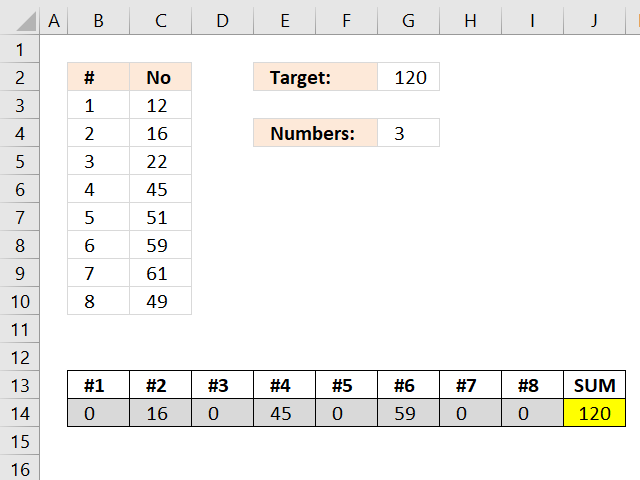Author: Oscar Cronquist Article last updated on February 14, 2019Excelxor is such a great website for inspiration, I am really impressed by this post Which numbers add up to total? (2): Multiple Solutions. The MMULT function is used in really clever way, I thought that function was pretty much useless.

I modified the formula in excelxor's post so it can identify the sum that is nearest to the target value, if there isn´t a total equal to target value.

The animated picture shows two target values in cell E1. The result is in cell range E6:L6 and the sum is in cell M6.

Array formula in cell range E6:L6:

=INDEX(MOD(INT((ROW(\$B\$2:INDEX(\$B:\$B, 2^ROWS(\$B\$2:\$B\$9)))-1)/2^(TRANSPOSE(MATCH(ROW(\$B\$2:\$B\$9), ROW(\$B\$2:\$B\$9)))-1)), 2)*TRANSPOSE(\$B\$2:\$B\$9), MATCH(MIN(ABS(MMULT(MOD(INT((ROW(\$B\$2:INDEX(\$B:\$B, 2^ROWS(\$B\$2:\$B\$9)))-1)/2^(TRANSPOSE(MATCH(ROW(\$B\$2:\$B\$9), ROW(\$B\$2:\$B\$9)))-1)), 2), \$B\$2:\$B\$9)-\$E\$1)), ABS(MMULT(MOD(INT((ROW(\$B\$2:INDEX(\$B:\$B, 2^ROWS(\$B\$2:\$B\$9)))-1)/2^(TRANSPOSE(MATCH(ROW(\$B\$2:\$B\$9), ROW(\$B\$2:\$B\$9)))-1)), 2), \$B\$2:\$B\$9)-\$E\$1), 0), 0)

### How do I enter this array formula?

1. Select cell range E6:L6
2. Paste the array formula in the formula bar
3. Press an hold CTRL + SHIFT
4. Press Enter
5. Release all keys

If you did it right the formula now begins and ends with curly brackets, like this {=array formula}. They appear automatically, don´t enter the curly brackets yourself.

### I want to use this formula, how do I change it?

The array formula uses this cell range \$B\$2:\$B\$9, if you need to work with more values like \$B\$2:\$B\$15 , you can change the formula following these steps.

1. Copy the formula to notepad
2. Press CTRL + H
3. Search string \$B\$2:\$B\$9
4. Replace string \$B\$2:\$B\$15
5. Click "Replace all" button
6. Copy the formula from notepad
7. Delete the old formula in excel
8. Enter the new array formula in E6:Q6, see steps above.

### How many numbers can I use?

If you have more than 15 numbers I recommend using my udf: Excel udf: Find positive and negative amounts that net to zero I think my udf can handle up to 20 maybe 22 values, it depends on your computer hardware.

Torstein made a great comment below: Is it possible to restrict solutions to those which have eg three numbers that adds to the total?
Yes, it is. See his question and my answer with a workbook you can download.

### I want to learn more about advanced formulas?

I have made an advanced excel course that is available online, check it out.

### Recommended reading

Which numbers add up to total? (2): Multiple Solutions

Which numbers add up to total?

Excel udf: Find positive and negative amounts that net to zero

Identify numbers in sum using solver

Find positive and negative amounts that net to zero

### Download *.xlsx file

Find numbers which are closest to a total.xlsx